# Modern Arabic mathematical notation

Modern Arabic mathematical notation

The designation modern Arabic mathematical notation is used for a mathematical notation based on the Arabic script that is widely used in the Arab world, especially at pre-university levels of education. Its form is mostly derived from Western notation, but has some notable features that set it apart from its Western counterpart. The most remarkable of those features is the fact that it is written from right to left following the normal direction of the Arabic script. Other differences include the replacement of the Latin alphabet letters for symbols with Arabic letters and the use of Arabic names for functions and relations.

## Some features of Arabic mathematical notation

• The most remarkable of those features is the fact that it is written from right to left following the normal direction of the Arabic script. Other differences include the replacement of the Latin alphabet letters for symbols with Arabic letters and the use of Arabic names for functions and relations.
• The notation exhibits one of the very few remaining vestiges of non-dotted Arabic scripts, as dots over and under letters (I'jam) are usually omitted.
• Letter cursivity (connectedness) of Arabic is also taken advantage of, in a few cases, to define variables using more than one letter. The most widespread example of this kind of usage is the canonical symbol for the radius of a circle نق (Arabic pronunciation: [nɑq]), which is written using the two letters nūn and qāf. When variable names are juxtaposed (as when expressing multiplication) they are written non-cursively.

## Arabic mathematical notation in different regions

The notation differs slightly from region to another. In university-level education most regions use the Western notation. The notation mainly differs in numeral system used, and in mathematical symbol used

### Numeral systems used in Arabic mathematical notation

There are three numeral systems used in right to left mathematical notation.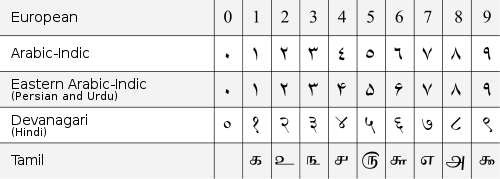### Arabic symbols and mirrored Latin symbols

• Sometimes, The symbols used in Arabic mathematical notation differs according to the region

e.g.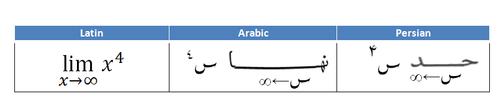• Sometimes, mirrored Latin symbols are used in Arabic mathematical notation (especially in western Arabic regions)

## Examples of Arabic mathematical notation

### Mathematical letters

• Usage of Latin x in maths is derived from the first letter of the Arabic word شيء [ʃajʔ(un)] meaning thing. (X was used in old Spanish for the sound /ʃ/).

### Sets and number systems

Description Latin Arabic
Natural numbers$\mathbb{N}$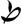Integers$\mathbb{Z}$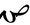Rational numbers$\mathbb{Q}$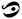Real numbers$\mathbb{R}$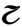Imaginary numbers$\mathbb{I}$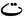Complex numbers$\mathbb{C}$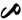Empty set$\varnothing$$\varnothing$
Is an element of$\in$$\ni$
Subset$\subset$$\supset$
Superset$\supset$$\subset$
Universal set$\mathbf{S}$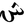### Arithmetic and algebra

Description Latin Arabic Notes
Decimal Point$\mathbf{.}$$\mathbf{,}$ a Decimal comma is used
Comma$\mathbf{,}$ ، ، is used instead of , in Latin notation
Percent  %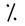Permille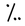Is proportional to$\propto$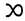n th root$^n\sqrt{\,\,\,}$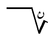Logarithm log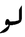Logarithm to base b log b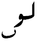Natural logarithm ln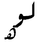Summation$\sum$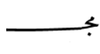also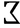in some regions
Product$\prod$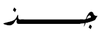also$\prod$ in some regions
factorial n!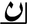also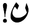in some regions
permutations$^n\mathbf{P}_r$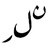also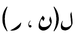is used in some regions as$\mathbf{P}(n,r)$
Combinations$^n\mathbf{C}_k$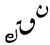also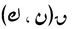is used in some regions as$\mathbf{C}(n,k)$
and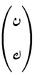as the binomial coefficient$n \choose k$

### Trigonometric and hyperbolic functions

#### Trigonometric functions

Latin Arabic Notes
sin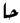also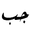is used in some regions (e.g. : Syria)
cos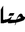also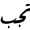is used in some regions (e.g. : Syria)
tan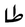also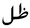is used in some regions (e.g. : Syria)
cot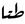also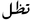is used in some regions (e.g. : Syria)
sec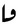csc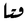#### Hyperbolic functions

The letter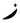is added to the end of trigonometric functions to express hyperbolic functions (the same way h is used in Latin notation).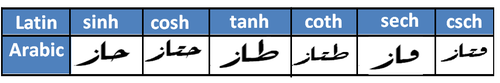#### Inverse trigonometric functions

The notation sin − 1 is the one used in Arabic notation for the inverse functions like: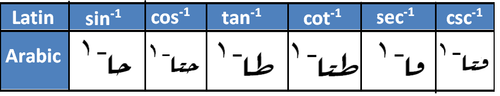#### Inverse hyperbolic functions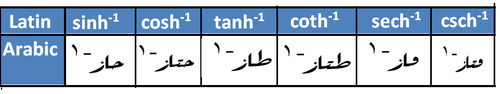### Calculus

Description Latin Arabic Notes
Limit lim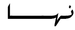also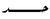is used in some regions (e.g. Iran)
function$\mathbf{f}(x)$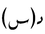derivatives$\mathbf{f'}(x), \dfrac{dy}{dx} , \dfrac{d^2y}{dx^2} , \dfrac{\partial {y}}{\partial{x}}$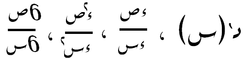Integrals$\int{} , \iint{} ,\iiint{}, \oint{}$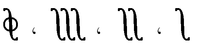### Complex analysis

Latin Arabic$z = x + iy = r(\cos{\varphi}+i \sin{\varphi})= r e^{i\varphi} = r\angle{\varphi}$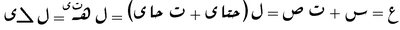Wikimedia Foundation. 2010.

Поможем написать курсовую

### Look at other dictionaries:

• Mathematical notation — For information on rendering mathematical formulas in Wikipedia, see Help:Formula. See also: Table of mathematical symbols Mathematical notation is a system of symbolic representations of mathematical objects and ideas. Mathematical notations are …   Wikipedia

• History of mathematical notation — Mathematical notation comprises the symbols used to write mathematical equations and formulas. It includes Hindu Arabic numerals, letters from the Roman, Greek, Hebrew, and German alphabets, and a host of symbols invented by mathematicians over… …   Wikipedia

• Notation (mathématiques) — Pour les articles homonymes, voir Notation. On utilise en mathématiques un ensemble de notations pour condenser et formaliser les énoncés et les démonstrations. Ces notations ce sont dégagées peu à peu au fil de l histoire des mathématiques et de …   Wikipédia en Français

• Notation — The term notation can refer to: Contents 1 Written communication 1.1 Biology and Medicine 1.2 Chemistry 1.3 Dance and movement …   Wikipedia

• Arabic numerals — The arabic numerals (often capitalized) are the ten digits (0, 1, 2, 3, 4, 5, 6, 7, 8, 9), which along with the system by which a sequence (e.g. 406 ) was read as a number were originally defined by Indian mathematicians, later modified and… …   Wikipedia

• Positional notation — Numeral systems by culture Hindu Arabic numerals Western Arabic (Hindu numerals) Eastern Arabic Indian family Tamil Burmese Khmer Lao Mongolian Thai East Asian numerals Chinese Japanese Suzhou Korean Vietnamese …   Wikipedia

• musical notation — Written, printed, or other visual representation of music. There are two basic approaches to notating music. Tablature (such as guitar chord diagrams) depicts the actions a performer is to take (in particular, showing where to put the fingers to… …   Universalium

• Integral — This article is about the concept of integrals in calculus. For the set of numbers, see integer. For other uses, see Integral (disambiguation). A definite integral of a function can be represented as the signed area of the region bounded by its… …   Wikipedia

• History of the Hindu-Arabic numeral system — The Hindu Arabic numeral system is a place value numeral system: the value of a digit depends on the place where it appears; the 2 in 205 is ten times greater than the 2 in 25. It requires a zero to handle the empty powers of ten (as in 205 ). [… …   Wikipedia

• Hindu–Arabic numeral system — Numeral systems by culture Hindu Arabic numerals Western Arabic (Hindu numerals) Eastern Arabic Indian family Tamil Burmese Khmer Lao Mongolian Thai East Asian numerals Chinese Japanese Suzhou Korean Vietna …   Wikipedia